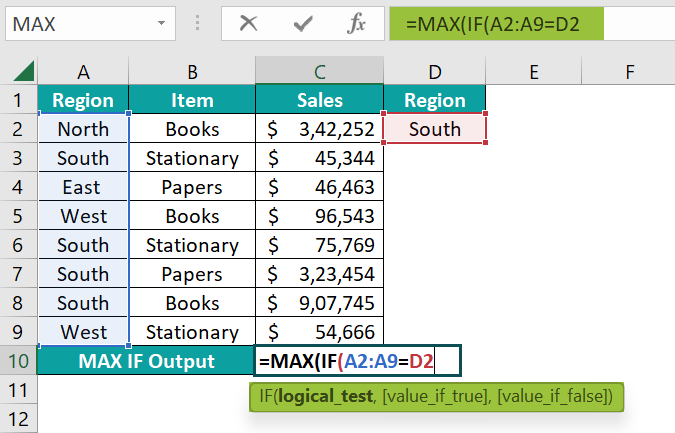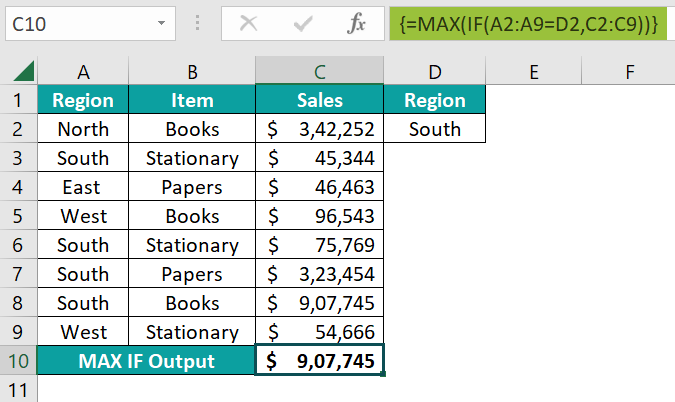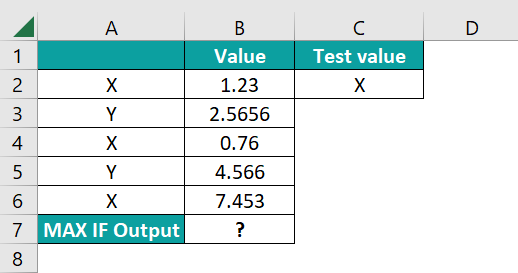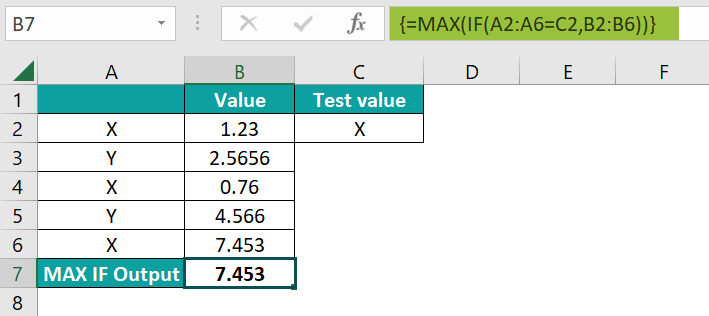# MAX IF In ExcelArticle byExcelMojo Team## What Is MAX IF In Excel?

The MAX IF in Excel is the combination of two Excel formula, MAX and IF, that matches the logical test conditions and identifies the maximum value from all the data. The MAX is an inbuilt Statistical function, and the IF is an inbuilt Logical function of the Functional library, so we can insert the formula or enter them directly in the worksheet.

For example, we will calculate the maximum value according to criteria using the MAX IF Function.

Select cell B7, enter the formula {=MAX(IF(A2:A6=C2,B2:B6))}, and pressEnter”.

The result is ‘50’, as shown above.

[Output Observation: First, the condition is checked in the cells A2 to A6, for cell values “A”, and in those cells, the maximum value is the output. Cells A2, A4, and A6 have the cell value A, and their corresponding cells, B2, B4, and B6, have values 10, 30, and 50, respectively. Therefore, cell A6 has the maximum value, i.e., 50.]

###### Key Takeaways
• The MAX IF in Excel identifies the maximum value from all the array values that match the logical test.
• The MAX function is an array formula that finds the maximum value in a given range.
• The IF function is a conditional function that displays results based on certain criteria.
• In the function, the logical test can run multiple times in a data set.
• The MAX IF formula is used to find the maximum marks obtained by a student, the maximum sales of a product, the maximum temperature of a month, etc.

### MAX IF() Excel Formula

The 2 inbuilt Excel formulas, i.e., MAX and IF, are combined to get the syntax of the MAX IF Excel Formula as follows:

=MAX(IF(criteria_range=criteria, max_range))

The arguments of the MAX IF Excel Formula are,

• criteria_range = criteria – It is a mandatory argument. It consists of two values: the criteria cell range of the dataset, and the second is the criteria, the value to be tested in the criteria range.
• max_range: It is a mandatory argument. It is the range of values from which the maximum value is to be fetched according to the criteria.

### How To Use MAX IF Excel Function?

We can use the MAX IF Excel Function in 2 ways, namely,

1. Access from the Excel ribbon.
1. MAX()
1. IF()
2. Enter in the worksheet manually.

Choose an empty cell → select the “Formulas” tab → go to the “Function Library” group → click the “More Functions…” option drop-down → click the “Statistical” option right arrow → select the “MAX” function, as shown below.

Choose an empty cell → select the “Formulas” tab → go to the “Function Library” group → click the “Logical” option drop-down → select the “IF” function, as shown below.

#### Method #2 – Enter in the worksheet manually

1. Select an empty cell for the output.
2. Type =MAX(IF()) in the selected cell. [Alternatively, type “=M” and double-click the MAX excel function from the list of suggestions, and then type “=I” and double-click the IF excel function from the list of suggestions shown by Excel.
3. Enter the arguments as cell references or cell values and press the “Enter” key.

Let us take an example to understand this function.

The succeeding example depicts the items and sales, and we will calculate the maximum value according to the criteria using the MAX IF in Excel.

In the table, the data is,

• Column A contains the Region.
• Column B contains the Item.
• Column C contains the Sales.
• Cell C10 contains the Output.

The steps to evaluate the values using the MAX IF formula are,

1. Select cell C10, and enter the formula =MAX(IF(A2:A9=D2, i.e., the ‘criteria_range’ as A2:A9, and the ‘criteria’ as “D2”.2. Enter the value of the ‘max_range’ as “C2:C9”, close the brackets and press the “Enter” key. The complete formula is {=MAX(IF(A2:A9=D2,C2:C9))} in cell C10.The output is “\$907,745”, as shown above.

### Examples

We will consider some advanced scenarios using the MAX IF in Excel examples.

#### Example #1

The succeeding example depicts the marks of girls and boys, and we will calculate the maximum value according to the criteria using the MAX IF in Excel.

In the table, the data is,

• Column A contains the Gender.
• Column B contains the Marks.
• Cell B7 contains the Output.

The procedure to evaluate the values using the MAX IF formula is,

Select cell B7, enter the formula {=MAX(IF(A2:A6=C2,B2:B6))}, and press the “Enter” key.

The result is “98”, as shown above.

#### Example #2

The succeeding example depicts the fruit cost of two years, and we will calculate the maximum value according to the criteria using the MAX IF in Excel.

In the table, the data is,

• Column A contains the Fruits.
• Column B contains the Year.
• Cell C6 contains the Output.

The procedure to evaluate the values using the MAX IF formula is,

Select cell C6, enter the formula {=MAX(IF(A2:A5=D2,C2:C5))}, and press the “Enter” key.

The result is “400”, as shown above.

#### Example #3

The succeeding example depicts the visitors according to the dates, and we will calculate the maximum value according to the criteria using the MAX IF in Excel.

In the table, the data is,

• Column A contains the Date.
• Column B contains the Visitors.
• Cell B10 contains the Output.

The procedure to evaluate the values using the MAX IF formula is,

Select cell B10, enter the formula {=MAX(IF(A2:A9=C2,B2:B9))}, and press the “Enter” key.

The result is “876”, as shown above.

### Important Things To Note

• We get the #VALUE! error, if we miss entering the function name within the brackets.
• If we miss signs like =, comma, or brackets, we will get the “#NAME?” error.

1. What does the MAX IF function do in Excel?

The combination of MAX and IF functions are used to find the maximum value under a given condition. This helps users find the maximum value from a given data range with specific criteria.
The syntax of the MAX IF function is {=MAX(IF(criteria_range=criteria,max_range))}

2. How does the MAX IF function work in Excel?

The Excel MAX IF Function works as follows:
1) Select an empty cell for the output.
2) Type =MAX(IF()) in the selected cell. [Alternatively, type “=M” and double-click the MAX function from the list of suggestions, and then type “=I” and double-click the IF function from the list of suggestions shown by Excel.
3) Enter the arguments as cell references or cell values and press the “Enter” key.

For example, suppose we have data and will calculate the maximum value according to criteria using MAX IF in Excel.The procedure to evaluate the values using the Excel MAX IF Function is,

Select cell B7, enter the formula {=MAX(IF(A2:A6=C2,B2:B6))}, andpress the “Enter” key.The result is “7.453”, as shown above.

3. What is the MAXIFS function in Excel?

The Excel MAXIFS Function returns the maximum value in a range that meets single or multiple criteria. The MAXIFS function is a built-in function in Excel that is categorized under a Statistical Function. We can use the logical operators like “>,<,>=,<=,<>,=”, and special characters like “* & ?” for partial matching.